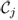8.4 Heuristic Candidate Selection Algorithm

A straightforward way to get the optimal Rj andto maximize the OEOT is to try all the ordered subsets offor each Rj, which runs in O(keN!) time, where k is the number of different rates, e is the base of natural logarithm, and N is the largest number of neighbors at all rates. It is, however, not feasible when N is large. In this section, we propose a heuristic algorithm to obtain a solution approaching the optimum.

As there is a finite number of transmission rates, a natural approach is to decompose the optimization problem into two parts. First, we find the optimal solution for each Rj; then, we pick the maximum one among them. So we only need to discuss how to find the solution approaching the optimum for a given rate, Rj, and the corresponding available next-hop neighbor set,. Lemma 8.1 guides us to design the heuristic algorithm.

Lemma 8.1 For given Rj and, defineas one feasible candidate set that achieves the maximum OEOT by selecting ...

Get Multihop Wireless Networks: Opportunistic Routing now with the O’Reilly learning platform.

O’Reilly members experience books, live events, courses curated by job role, and more from O’Reilly and nearly 200 top publishers.